# The chemical formula for hydrogen chloride is HCI. A chemist determined by measurements that 0.015 moles...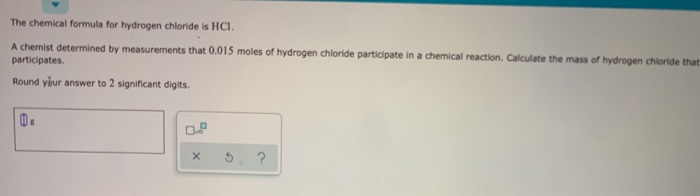The chemical formula for hydrogen chloride is HCI. A chemist determined by measurements that 0.015 moles of hydrogen chloride participate in a chemical reaction. Calculate the mass of hydrogen chloride that participates Round your answer to 2 significant digits. x S. ?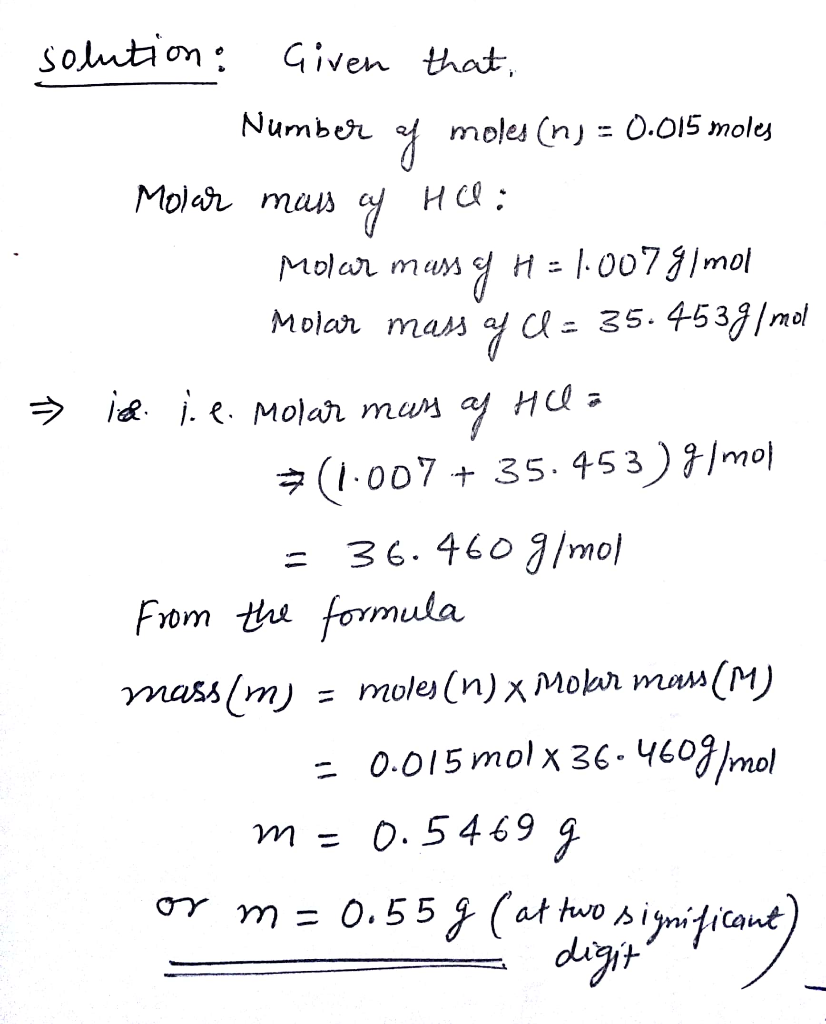##### Add Answer of: The chemical formula for hydrogen chloride is HCI. A chemist determined by measurements that 0.015 moles...
Similar Homework Help Questions
• ### A chemist determined by measurements that 0.0800 moles of potassium participated in a chemical reaction. Calculate...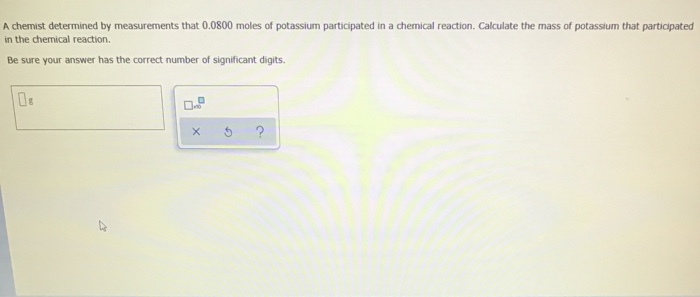A chemist determined by measurements that 0.0800 moles of potassium participated in a chemical reaction. Calculate the mass of potassium that participated in the chemical reaction. Be sure your answer has the correct number of significant digits. 070 x 5 ?

• ### O CHEMICAL REACTIONS Calculating molarity using solute moles A chemist prepares a solution of aluminum chloride...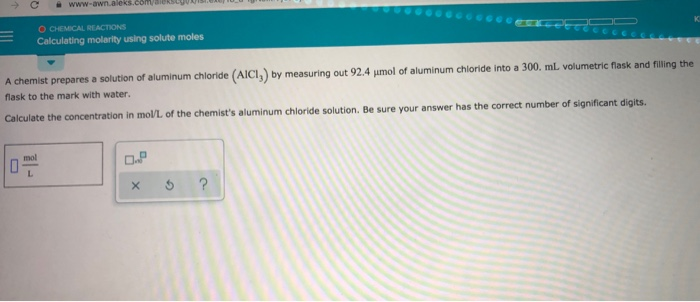O CHEMICAL REACTIONS Calculating molarity using solute moles A chemist prepares a solution of aluminum chloride (AICI) by measuring out 92.4 mol of aluminum chloride into a 300. mL volumetric flask and filling the flask to the mark with water. Calculate the concentration in mol/L of the chemist's aluminum chloride solution. Be sure your answer has the correct number of significant digits. 0

• ### Aqueous hydrochloric acid (HCI) will react with solid sodium hydroxide (NaOH) to produce aqueous sodium chloride (NaCl)...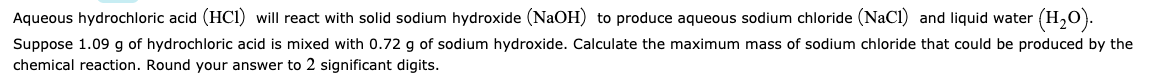Aqueous hydrochloric acid (HCI) will react with solid sodium hydroxide (NaOH) to produce aqueous sodium chloride (NaCl) and liquid water (H20). Suppose 1.09 g of hydrochloric acid is mixed with 0.72 g of sodium hydroxide. Calculate the maximum mass of sodium chloride that could be produced by the chemical reaction. Round your answer to 2 significant digits.

• ### Aqueous hydrochloric acid (HCI) will react with solid sodium hydroxide (NaOH) to produce aqueous sodium chloride...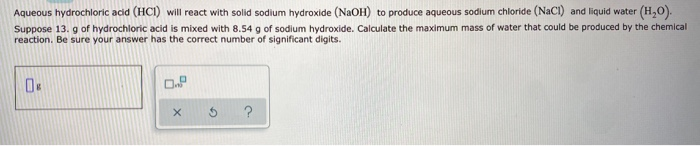Aqueous hydrochloric acid (HCI) will react with solid sodium hydroxide (NaOH) to produce aqueous sodium chloride (NaCl) and liquid water (H,0). Suppose 13. g of hydrochloric acid is mixed with 8.54 g of sodium hydroxide. Calculate the maximum mass of water that could be produced by the chemical reaction. Be sure your answer has the correct number of significant digits. 04 0. X 5 ?

• ### O RE BEACTIONS Solving a redox sitration problem To measure the amount of calcium carbonate (CaCO)...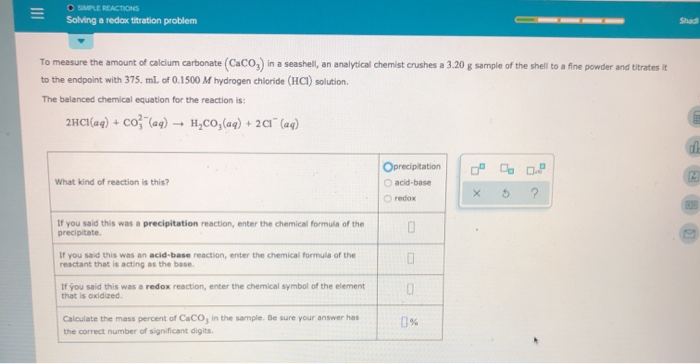O RE BEACTIONS Solving a redox sitration problem To measure the amount of calcium carbonate (CaCO) in a seashell, an analytical Chemist crushes a 3.20 g sample of the shell to a fine powder and titrates it to the endpoint with 375. ml. of 0.1500 M hydrogen chloride (HCI) solution. The balanced chemical equation for the reaction is: 2HCl(aq) + Co (aq) → H2CO3(aq) + 2a" (aq) What kind of reaction is this? Oprecipitation acid-base redox If you said this...

• ### e Shenon at is the difference between hydrogen chloride and hydrochloric acid? Hydrogen chloride is a...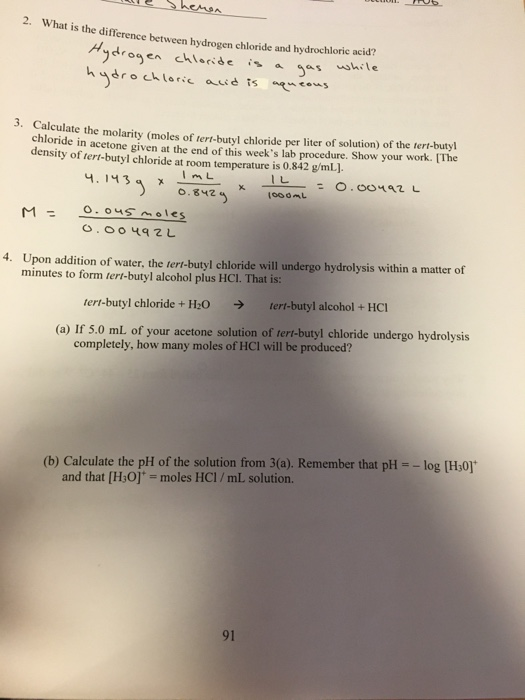e Shenon at is the difference between hydrogen chloride and hydrochloric acid? Hydrogen chloride is a gas while hydrochloric acid is aqueous 3. Calcul Calculate the molarity (moles of tert-butyl chloride per liter of solution) of the tert-butyl chloride in acetone given at the end of this week's lab procedure. Show your work. The density of tert-butyl chloride at room temperature is 0.842 g/mL). - = 0.00492 L ) 0.842 100ML 9.1439 x ImL * IL 0.842 M = o.ous...

• ### Ammonia will decompose into nitrogen and hydrogen at high temperature. An industrial chemist stud...

Ammonia will decompose into nitrogen and hydrogen at high temperature. An industrial chemist studying this reaction fills a 5.0 L flask with 1.3 atm of ammonia gas at 46. °C . He then raises the temperature, and when the mixture has come to equilibrium measures the amount of nitrogen gas to be 0.39 atm . Calculate the pressure equilibrium constant for the decomposition of ammonia at the final temperature of the mixture. Round your answer to 2 significant digits. kp=_______

• ### A chemist adds 305.0mL of a 0.32/molL copper(II) sulfate CuSO4solution to a reaction flask. Calculate the...

A chemist adds 305.0mL of a 0.32/molL copper(II) sulfate CuSO4solution to a reaction flask. Calculate the mass in grams of copper(II) sulfate the chemist has added to the flask. Round your answer to 2 significant digits.

• ### The reaction of hydrogen chloride(g) with ammonia(g) to form ammonium chloride(s) proceeds as follows. HCI(g) +...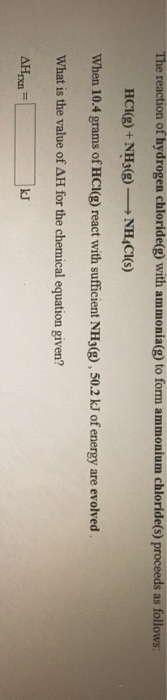The reaction of hydrogen chloride(g) with ammonia(g) to form ammonium chloride(s) proceeds as follows. HCI(g) + NH3(g) - NHACI(S) When 10.4 grams of HCl(g) react with sufficient NH3(g), 50.2 kJ of energy are evolved What is the value of AH for the chemical equation given? АНrxn=

• ### A chemist weighed out 101. g of iron. Calculate the number of moles of iron she...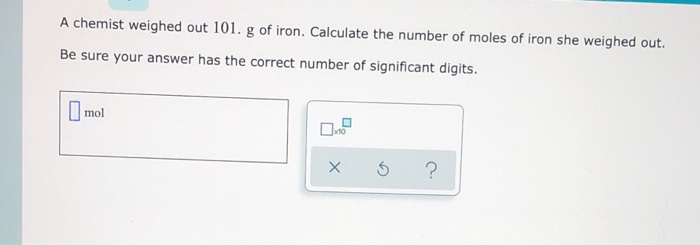A chemist weighed out 101. g of iron. Calculate the number of moles of iron she weighed out. Be sure your answer has the correct number of significant digits. mol x 5 ?

Need Online Homework Help?